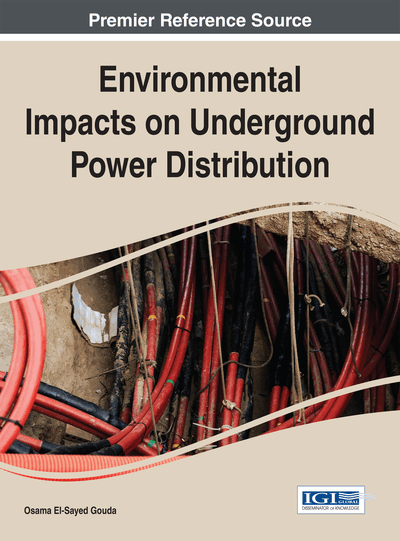# De-Rating Factor Due to the Dry-Band Formation

DOI: 10.4018/978-1-4666-6509-5.ch007
Available
\$33.75
List Price: \$37.50
10% Discount:-\$3.75
TOTAL SAVINGS: \$3.75

## Abstract

This chapter investigated the factors affecting the cable loading such as dry band formation, conductor temperature, sheath temperature, cable jacket temperature and exterior duct temperature and de-rating factor for different soil under testing. Applications are carried out using different ratings of distribution cables.
Chapter Preview
Top

## 7.1 De-Rating Factor Definition And Calculation

Formation of dry zones around the power cables which affect the cable loading is reported by KEMA Report. (1981) and Koopmans, G., & Gouda, O. E. (1985).

By De-rating Factor we mean the ratio between current ampacity of the cable with dry band formation and the cable ampacity assuming there is no dry band is formed.

Publication of IEC 60 287-1-3 (1982) gives formula to calculate the maximum current carrying capacity of underground power cables without taking the dry band into consideration as given in equation 1

(1)

In IEC 60 287-1-3 (1982) the dry band formation is considered in the calculations of maximum current carrying capacity of the underground power cables according to the following formulas:

(2)

Where:=is the difference between the conductor temperatureand the ambient temperatureoC, number of load carrying conductors in the cable is n (they are of equal size and carrying the same load),is the Dielectric loss per unit length for the insulation surrounding the conductor per phase,is the Alternating current resistance per unit length of the conductor at its maximum operating temperature (,is the Thermal resistance per unit length per core between conductor and sheath (oC.m/W),is the Thermal resistance per unit length of bedding between sheath and armour (oC.m/W),is the Thermal resistance per unit length of the external serving of the cable (Com/w),is the Thermal resistance per unit length between the cable surface and the surrounding soil (oC.m/W),is the Ratio of losses in the metal sheath to total losses in all conductors in that cable, andis the Ratio of armouring losses to conductors total losses.

To calculate the maximum current carrying capacity of the underground power cables or the cable ampacity in case of dry band formation the ratio between the dry and moist gives resistivites of the backfill soil () and the difference between the critical temperature of boundary between the moist and the dry zones inand ambient temperature () have to be obtained.

## Complete Chapter List

Search this Book:
Reset